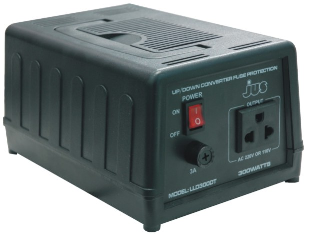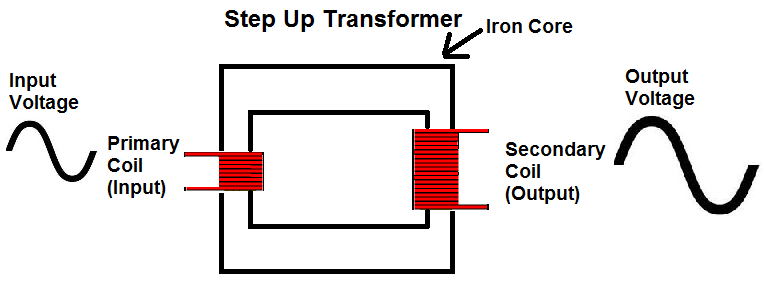﻿ What is a Step-Up Transformer?

# What is a Step-up Transformer?A Step-up Transformer is an AC-AC converter which converts the input AC signal into an AC signal with a larger voltage.A step-up transformer acts as a voltage-increasing device. The amount by which it increases the input voltage depends on the ratio of the the number of turns in the primary coil to the number of turns in the secondary coil.

If, for example, the secondary coil has double the amount of turns as the primary coil, the ratio will be 1:2 and the output voltage will be double the input voltage.

Though step-up transformers increase the voltage of the output voltage, it comes at a price. Transformers are simply conversion devices. They do not create voltage or power. So if a step-up transformer increases voltage, it decreases current. If it doubles the voltage output, the current output gets cut in half. So that the output signal now has half the current capability as the input signal. Step-up transformers never create power; they only convert it into different forms.